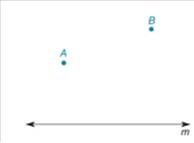Chapter 3.3, Problem 16E### Elementary Geometry for College St...

6th Edition
Daniel C. Alexander + 1 other
ISBN: 9781285195698

#### Solutions

Chapter
Section### Elementary Geometry for College St...

6th Edition
Daniel C. Alexander + 1 other
ISBN: 9781285195698
Textbook Problem
10 views

# In Exercises 13 to 18, describe the line segments as determined, undetermined, or overdetermined. Use the accompanying drawing for reference.Draw a line segment A B ¯ perpendicular to m .

To determine

To draw:

The line segment AB¯ perpendicular to line m and describe it as determined, underdetermined, or over-determined.

Explanation

Given:

The given figure is as follows.

Figure (1)

Approach:

If there is no possibility for occurrence of a line segment, it is considered as over determined segment

Calculation:

Consider the given figure.

Figure (2)

From figure (2), it can be analyzed only one line with end point A and B can be drawn which is not perpendicular to line m

### Still sussing out bartleby?

Check out a sample textbook solution.

See a sample solution

#### The Solution to Your Study Problems

Bartleby provides explanations to thousands of textbook problems written by our experts, many with advanced degrees!

Get Started

#### Solving an Equation In Exercises 722, solve for x. e3x=e4

Calculus: Early Transcendental Functions (MindTap Course List)

#### f(x)=1x2+1; limxf(x); and limxf(x) x 1 10 100 1000 f(x) x 1 10 100 1000 f(x)

Applied Calculus for the Managerial, Life, and Social Sciences: A Brief Approach

#### Using for |x| < 1,

Study Guide for Stewart's Multivariable Calculus, 8th

#### Express 198 inches as feet.

Mathematics For Machine Technology